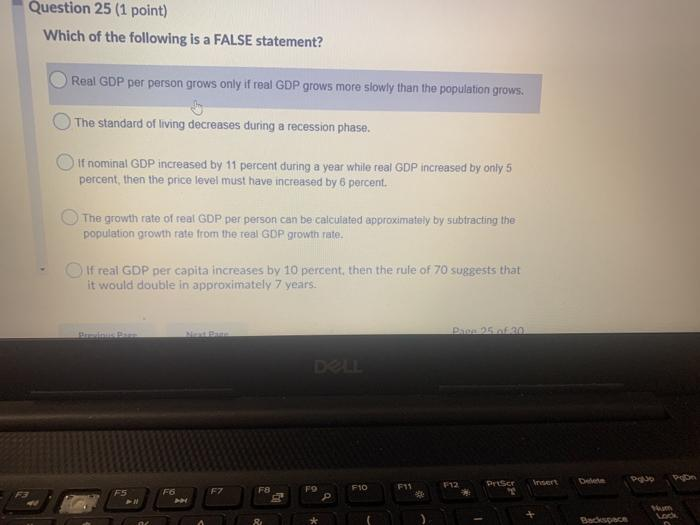### Create an Account

Home / Questions / Which of the following is a FALSE statement? Real GDP per person grows only if real GDP gr...

# Which of the following is a FALSE statement? Real GDP per person grows only if real GDP grows more slowly than the population grows. The standard of living decreases during a recession phase

Which of the following is a FALSE statement? Real GDP per person grows only if real GDP grows more slowly than the population grows. The standard of living decreases during a recession phase. if nominal GDP increased by 11 percent during a year while real GDP increased by only 5 percent, then the price level must have increased by 6 percent. The growth rate of real GDP per person can be calculated approximately by subtracting the population growth rate from the real GDP growth rate. If real GDP per capita increases by 10 percent, then the rule of 70 suggests that it would double in approximately 7 years. Date 20 DLL et F12 Priser De pas F10 F11 F6 F8 F7 FS PN FO P 8 BKSMay 11 2021 View more View LessSubscribe To Get Solution